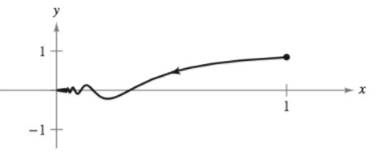Chapter 10, Problem 12PS

Chapter
Section
Textbook Problem

Arc Length A particle is moving along the path described by the parametric equations x = 1 t   and   y = sin t t for 1 ≤ t < ∞ , as shown in the figure. Find the length of this path.To determine

To calculate: The length of the path x=1t,y=sintt.

Explanation

Given:

The path is described from the following parametric equations where t starts from 1 and keeps on increasing indefinitely.

x=1ty=sintt

Formula Used:

The arc length for a parametric curve defined by the equation x(t) and y(t) where t varies between a and b is given by the equation:

A=ab(x'(t))2+(y'(t))2dt

Calculation:

For the provided parametric curve:

x(t)=1tx'(t)=1t2y(t)=sintty'(t)=tcostsintt2

This gives the arc length as:

A=

Still sussing out bartleby?

Check out a sample textbook solution.

See a sample solution

The Solution to Your Study Problems

Bartleby provides explanations to thousands of textbook problems written by our experts, many with advanced degrees!

Get Started

In Exercises 516, evaluate the given quantity. log1,000

Finite Mathematics and Applied Calculus (MindTap Course List)

In Exercises 75-98, perform the indicated operations and/or simplify each expression. 85. (x + 8)(x 2)

Applied Calculus for the Managerial, Life, and Social Sciences: A Brief Approach

Find each value. logaa

College Algebra (MindTap Course List)